# Riesz potential

(diff) ← Older revision | Latest revision (diff) | Newer revision → (diff)-potential

A potential of the formwhereis a positive Borel measure of compact support on the Euclidean space,, and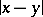is the distance between the points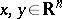. Whenand, the Riesz potential coincides with the classical Newton potential; whenand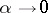, the limit case of the Riesz potential is in some sense the logarithmic potential. Whenand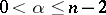, the Riesz potential is a superharmonic function on the entire space; moreover, in the classical case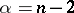, outside the support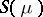of, the potential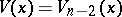is a harmonic function. When, the Riesz potential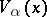is a subharmonic function outside. For allthe Riesz potentialis a lower semi-continuous function on, continuous outside.

Among the general properties of Riesz potentials the following are the most important. The continuity principle: Ifand if the restrictionis continuous at the point, thenis continuous atas a function on. The restricted maximum principle: If, then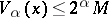everywhere on. When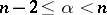, a more precise maximum principle is valid: If, then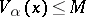everywhere on(this statement remains valid also whenand, that is, for the logarithmic potential).

The capacity theory for Riesz potentials can be constructed, for example, on the basis of the concept of the-energy of a measures: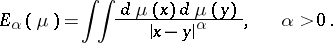One may assume that for a compact set,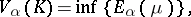where the infimum is taken over all measuresconcentrated onand such that; then the-capacity is equal toIf, then the infimum is attained on the capacitary measure(also called equilibrium measure), which is concentrated on,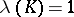, generating the corresponding capacitary-potential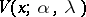(cf. also Capacity potential). The further construction of-capacities of arbitrary sets is carried out in the same way as for the classical capacities.

The Riesz potential is called after M. Riesz (see ), who obtained a number of important properties of Riesz potentials; for the first time such potentials were studied by O. Frostman (see ).

How to Cite This Entry:
Riesz potential. Encyclopedia of Mathematics. URL: http://encyclopediaofmath.org/index.php?title=Riesz_potential&oldid=14705
This article was adapted from an original article by E.D. Solomentsev (originator), which appeared in Encyclopedia of Mathematics - ISBN 1402006098. See original article